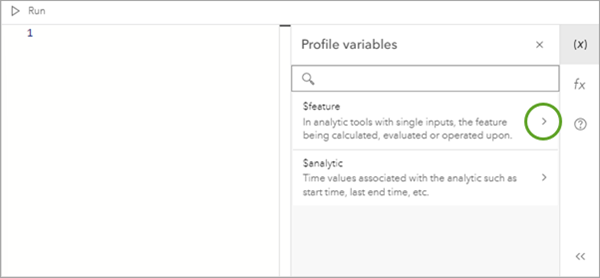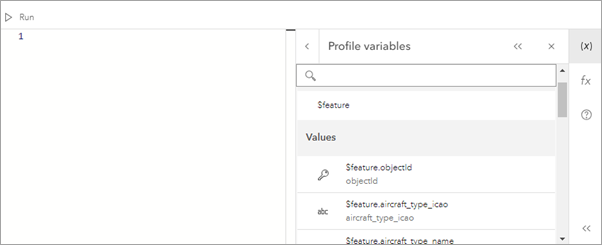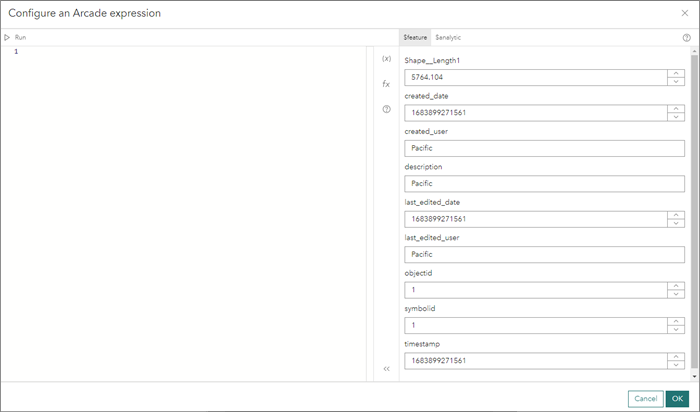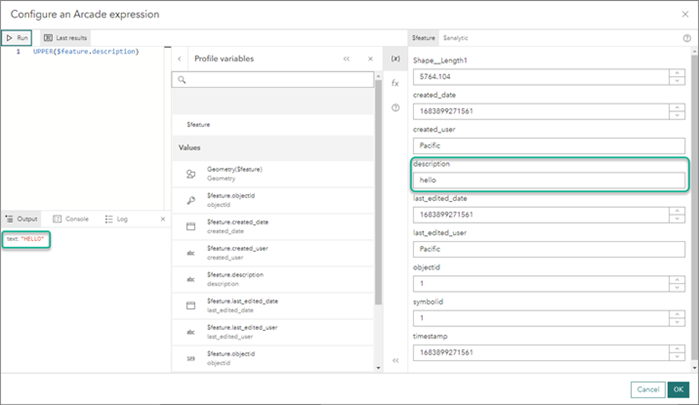ArcGIS Arcade is a portable, lightweight, and secure expression language that can perform mathematical calculations, manipulate text, and evaluate logical statements. It also supports multi-statement expressions, variables, and flow control statements. What makes Arcade particularly unique to other expression and scripting languages is its inclusion of feature and geometry data types. A common use case for Arcade is to perform a calculation with layer fields and geometry.

## Usage notes

Keep the following in mind when using Arcade expressions:

• Arcade expressions are supported in the Detect Incidents, Calculate Field, Filter by Expression, Map Fields, Join Features, Create Buffers, and Reconstruct Tracks tools.
• Expressions may incorporate attributes from input feeds and sources as well as values that describe the analytic in which the expression is configured.
• In the expression builder, the profile variables can be expanded to display a list of fields, time values, target fields, and join fields. ArcGIS Velocity expressions leverage the following profile variables:
• \$feature—In all tools, except Join Features, this variable contains the attribute fields of the incoming features from feeds and sources. Expressions can be built that leverage feature attribute values by selecting fields from this variable.
• \$analytic—This variable contains values specific to the analytic such as \$analytic.AnalyticStartTime and \$analytic.AnalyticLastEndTime.
• In order to convert these analytic variable values from an epoch integer (1646409900000) to a date, use the Arcade Date() function. For details, see Date functions in the ArcGIS Arcade documentation.
• \$target—In the Join Features tool, for the Join Condition expression, this profile variable contains the attribute fields from the target feed or source.
• \$join—In the Join Features tool, for the Join Condition expression, this profile variable contains the attribute fields from the join source.
• Access fields and schema components in the Arcade expression builder.
• From the Profile variables pane, access fields, variables, and components of the schema related to the category, for example, \$feature, \$analytic, \$target, or \$join.• This displays the fields or variables related to that variable.• Arcade provides default sampled values for each tool depending on the incoming schema. You can update the sampled values while building the expressions for each tool.
• To adjust sample values for Arcade expression testing, do the following:
• Based on the incoming schema, if you click to edit an Arcade expression, the Configure an Arcade expression window opens.
• On the \$feature tab, notice that for each incoming field, there is a sampled value displayed based on its data type. For example, every string field has a sample value of Pacific. To build and test a valid expression for different datasets, you must change the sampled values provided for a field so the Arcade expression will evaluate the expression and return the correct data type returned by the expression.• Adjust the sampled value if used in Arcade expression testing and evaluation.• Click Run in the upper left of the Configure an Arcade expression window to run the Arcade expression. The expression will evaluate based on the updated sampled data provided.
• Build Arcade expressions to handle null and 0.
• In mathematic operations, the Arcade expression language treats null values as 0 for evaluation. Therefore, Arcade mathematical expressions in ArcGIS Velocity should be created to account for null values in your data.
• Account for null values in your data by handling null values. Consider the expression \$feature.DistanceToFeature <= 5. This expression should be built in the Arcade language to handle nulls such as \$feature.DistanceToFeature != null && \$feature.DistanceToFeature <= 5. In these expressions, the <= 5 is a mathematic operation and if the DistanceToFeature field value is null, it is evaluated as 0, which would pass the expression.

## Mathematical operator and function examples

Expressions can mathematically process numbers. The table below provides a sample of available operations. For more information about mathematical operations in Arcade, see Mathematical Functions.

 Operator Explanation Example Result a + b a plus b. fieldname contains a value of 1.5 \$target["fieldname"] + 2.5 4.0 a - b a minus b. fieldname contains a value of 3.3 \$target["fieldname"]- 2.2 1.1 a * b a times b. fieldname contains a value of 2.0 \$join["fieldname"] * 2.2 4.4 a / b a divided by b. fieldname contains a value of 4.0 \$join["fieldname"] / 1.25 3.2 abs( a ) Returns the absolute (positive) value of a. fieldname contains a value of -1.5 abs(\$target["fieldname"]) 1.5 log( a ) Returns the natural logarithm (base E) of a. fieldname contains a value of 1 log(\$join["fieldname"]) 0 sin( a ) Returns the trigonometric sine of a. The input is assumed to be an angle in radians. fieldname contains a value of 1.5707 sin(\$target["fieldname"]) 1 cos( a ) Returns the trigonometric cosine of a. The input is assumed to be an angle in radians. fieldname contains a value of 0 cos(\$join["fieldname"]) 1 tan( a ) Returns the tangent of a. The input is assumed to be an angle in radians. fieldname contains a value of 0 tan(\$target["fieldname"]) 0 sqrt( a ) Returns the square root of a. fieldname contains a value of 9 sqrt(\$join["fieldname"]) 3 min( a, b ) Returns the lowest-valued number between a and b. fieldname contains a value of 1.5 and a value of -3 min(\$join["fieldname"], -3) -3 max( a, b ) Returns the highest-valued number between a or b. fieldname1 contains a value of 1.5 and fieldname2 contains a value of -3 max(\$target["fieldname1"], \$join["fieldname2"]) 1.5 constrain(,,) Returns the input value if it is within the constraining bounds. If the input value is less than the low value, it returns the low value. If the input value is greater than the high value, it returns the high value. constrain(\$target["distance"], 0, 10) constrain(\$join['Store dist'], 6, distance) Returns 0 if distance is less than 0, 10 if distance is greater than 10, and distance otherwise. Returns 6 if Store dist is less than 6, distance if Store dist is greater than distance, and Store dist otherwise.

The following multiplication example for a join condition expression uses a field from the target dataset:

\$target["Distance"] * 2 > \$join["DistField"]

## Text function examples

 Operator Explanation Example Result concatenate(, ) Concatenates values together and returns a string. values—An array of string values to concatenate.separator (optional)—A separator to use for concatenation if the values parameter is an array or a string to concatenate if a single value is provided for the first parameter. If not provided, it will be empty. fieldname contains a value of ArcGIS Velocity concatenate([\$target["fieldname"], "is", "great!"], ' ') ArcGIS Velocity is great! find(, , ) Finds a string within a string. Wildcards are not supported.searchText—The substring to search for.text—The text to search.startPos (optional)—The zero-based index of the location in the string to search from. fieldname1 contains a value of 14NorthStreet and fieldname2 contains a value of North find(\$target["fieldname2"], \$join["fieldname1"]) 2 lower() Makes a string lowercase.value - The string to be made lowercase. fieldname contains a value of ANALYTICS lower(\$join["fieldname"]) analytics

The following text example uses find and lower:

find(("north"), lower("146NorthStreet")) == False

## Date function examples

Arcade can process dates. In Arcade, month values range from 0 (January) to 11 (December), days from 1 to 31, hours from 0 (12:00 a.m.) to 23 (11:00 p.m.), minutes and seconds from 0 to 59, and milliseconds from 0 to 999.

 Operator Explanation Example Result date( , , , , ) Parses a value or set of values into a date string.value (optional)—Either the number of milliseconds since January 1, 1970, UTC or a number representing a year. If a year is specified, the month and day must also be provided in subsequent parameters. This value may also be a date string or an ISO 8601 string to be converted into a date.month (optional)—The month (0-11), where 0 is January and 11 is December.day (optional)—The day of the month (1-31).hour (optional)—The hour of the day (0-23).minute (optional)—The minute of the hour (0-59).second (optional)—The second of the minute (0-59).millisecond (optional)—The millisecond of the second (0-999). fieldname contains a value of 1476987783555 Example 1: Date(\$target["fieldname"]) Example 2: Date(2017,0,14,0) Example 3: Date() Example 1: 20 Oct 2016 11:23:03 am Example 2: 14 Jan 2017 12:00:00 am Example 3: Returns the current time DateDiff(, , ) Subtracts two dates and returns the difference in the specified units.date1—The date value from which to subtract a second date.date2—The date value to subtract from the first given date.startpos (optional)—The units in which to return the difference of the two given dates. The supported unit types include milliseconds, seconds, minutes, hours, days, months, and years. The default value is milliseconds. Example 1: DateDiff(Date(2017,1,14,0), Date()) Example 2: DateDiff(Date(2017,1,14,0), Date(), "Years") Result will vary depending on when the command is run. Example 1: -20532129137 Example 2: -0.6546783768647119 Year() Returns the year of the given date. value—A date value from which to get the year. Example 1: fieldname is a field of type Date with a value of value of 09 Oct 2017 04:30:43 pm Year(\$join["fieldname"]) Example 2: fieldname is a string field formatted as an ISO 8601 string with a value of 2012-09-27 Example 3: fieldname is a string field formatted as an ISO 8601 string with a value of Year(Date(\$target["fieldname"])) Example 1: 2017 Example 2: 2012

## Conditional operators

Conditional statements can use the following operators:

 Operator Explanation Example Results a > b a < b a is greater than b. a is less than b. 10 > 2 False a >= b a <= b a is greater than or equal to b. a is less than or equal to b. abs(-10) >= 10 True a != b a is not equal to b. abs(-3) != -3 True a == b a is equal to b. abs(-5) == 5 True || Condition 1 or condition 2 is met. (abs(-5) == 5) || (10 < 2) True && Condition 1 and condition 2 are met. (abs(-5) == 5) && (10 < 2) False

The following example of a buffer expression uses advanced functions and conditions:

iif(field1 > field2, iif(field2 = 0, field3, field4), 0)

The following is a multiplication example for a join condition:

iif(field1 > field2, iif(field2 = 0, field3, field4), 0) > \$join["Distance"] * 2